JEE  >  BITSAT Physics Test - 3

# BITSAT Physics Test - 3

Test Description

## 40 Questions MCQ Test BITSAT Mock Tests Series & Past Year Papers | BITSAT Physics Test - 3

BITSAT Physics Test - 3 for JEE 2023 is part of BITSAT Mock Tests Series & Past Year Papers preparation. The BITSAT Physics Test - 3 questions and answers have been prepared according to the JEE exam syllabus.The BITSAT Physics Test - 3 MCQs are made for JEE 2023 Exam. Find important definitions, questions, notes, meanings, examples, exercises, MCQs and online tests for BITSAT Physics Test - 3 below.
Solutions of BITSAT Physics Test - 3 questions in English are available as part of our BITSAT Mock Tests Series & Past Year Papers for JEE & BITSAT Physics Test - 3 solutions in Hindi for BITSAT Mock Tests Series & Past Year Papers course. Download more important topics, notes, lectures and mock test series for JEE Exam by signing up for free. Attempt BITSAT Physics Test - 3 | 40 questions in 50 minutes | Mock test for JEE preparation | Free important questions MCQ to study BITSAT Mock Tests Series & Past Year Papers for JEE Exam | Download free PDF with solutions
 1 Crore+ students have signed up on EduRev. Have you?
BITSAT Physics Test - 3 - Question 1

### A current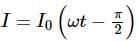flows in an a.c. circuit. An e.m.f. E − E0 sin ω t is applied to it. Then the power consumption P is

Detailed Solution for BITSAT Physics Test - 3 - Question 1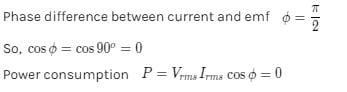BITSAT Physics Test - 3 - Question 2

### The control rod in a nuclear reactor is made of

BITSAT Physics Test - 3 - Question 3

### The nuclear reactor at Kaiga is a

BITSAT Physics Test - 3 - Question 4

Two wires of the same dimensions but resistivities ρ₁ and ρ₂ are connected in series. The equivalent resistivity of the combination is

BITSAT Physics Test - 3 - Question 5

A potential difference of V is applied at the ends of a copper wire of length l and diameter d. On doubling only d, drift velocity

BITSAT Physics Test - 3 - Question 6

If intensity of incident light is increased in PEE then which of the following is true

BITSAT Physics Test - 3 - Question 7

The de Broglie wavelength associated with the particle of mass m moving with velocity v is

BITSAT Physics Test - 3 - Question 8

The current flowing in a coil of self inductance 0.4 mH is increased by 250 mA in 0.1 s. The e.m.f. induced will be

Detailed Solution for BITSAT Physics Test - 3 - Question 8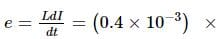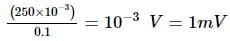As current is increasing, induced e.m.f. is in opposite direction, therefore, e = - 1 mV.

BITSAT Physics Test - 3 - Question 9

A capacitor of capacitance 1 μ F is charged to 1KV. The energy stored in the capacitor in joules is

Detailed Solution for BITSAT Physics Test - 3 - Question 9

C = 1μF
V = 1KV
energy stored U = 1/2 (CV2)
= 1/2 * 1 * 12 = 0.5

BITSAT Physics Test - 3 - Question 10

When we touch the terminals of a high voltage capacitor, even after a high voltage has been cut off, then the capacitor has a tendency to

BITSAT Physics Test - 3 - Question 11

It is easier to roll a barrel than pull it along the road. This statement is

BITSAT Physics Test - 3 - Question 12

If radius of the earth contracted 2% and its mass remains the same then weight of the body at the earth surface

BITSAT Physics Test - 3 - Question 13

A black body radiates 20J of energy at 227oC temperature.If temperature of the black body is changed to 727oC, then energy radiated will be

BITSAT Physics Test - 3 - Question 14

A gas at certain temperature and volume has a pressure equal to 75 cm of Hg. If the mass of the gas is doubled, at the same volume and same temperature, its new pressure will be

BITSAT Physics Test - 3 - Question 15

The magnetic field at the centre of a circular coil of radius r carrying current I is B₁. The field at the centre of another coil of radius 2 r carrying same current I is B₂. The ratio B₁/B₂ is

BITSAT Physics Test - 3 - Question 16

When a diamagnetic substance is brought near north or south pole of a bar magnet is

BITSAT Physics Test - 3 - Question 17

Spherical balls of radius R are falling in a viscous fluid of viscosity η with a velocity v. The retarding viscous force acting on the spherical ball is

BITSAT Physics Test - 3 - Question 18

Rain drops are spherical in shape, because of

BITSAT Physics Test - 3 - Question 19

A wire of length L and radius a rigidly fixed at one end. On stretching the other end of the wire with a force F, the increase in it length is l. If another wire of same material but of length 2L and radius 2a is stretched with a force 2F, the increase in its length will be

BITSAT Physics Test - 3 - Question 20

Water drops fall at regular intervals from a tap which is 5 m above the ground. The third drop is leaving the tap at the instant the first drop touches the ground. How far above the ground is the second drop at that instant

Detailed Solution for BITSAT Physics Test - 3 - Question 20
Given:
Height =h=5m
To calculate time taken by first drop to reach ground:
u=0m/s, t=T
so,
s=ut+1/2at^2
5=0+1/2aT^2-----(1)
Time taken by second drop will be T/2
Therefore, its distance from tap
h=0+1/2(aT^2)/4       ------------(2)
divide equ (ii) by equ (1)
h/5=1/4
h=1.25m
therefore H-h=5-1.25=3.75m

BITSAT Physics Test - 3 - Question 21

A circular road of radius 1000 m has banking angle 45o. The maximum safe speed of a car having mass 2000 kg will be, if the coefficient of friction between tyre and road is 0.5

BITSAT Physics Test - 3 - Question 22

The distance travelled by an object along the axes are given by x = 2t2, y = t2 - 4t, z = 3t - 5. The initial velocity of the particle is

BITSAT Physics Test - 3 - Question 23

A body of mass 2 kg is hung on a spring balance mounted vertically in a lift. If the lift descends with an acceleration equal to the acceleration due to gravity 'g', the reading on the spring balance will be

BITSAT Physics Test - 3 - Question 24

The hypermetropia is a

BITSAT Physics Test - 3 - Question 25

A body is executing simple harmonic motion with an angular frequency 2 rad/s. The velocity of the body at 20 mm displacement, when the amplitude of motion is 60 mm, is

BITSAT Physics Test - 3 - Question 26

If a spring extends by x on loading, then the energy stored by the spring is (if T is tension in the spring and k is spring constant)

BITSAT Physics Test - 3 - Question 27

Two plane mirrors are inclined at an angle θ . It is found that a ray incident on one mirror at any angles is rendered parallel to itself after reflection from both the mirrors. The value of θ is

BITSAT Physics Test - 3 - Question 28

Of two eggs which have identical sizes, shapes and weights, one is raw and the other is half-boiled. The ratio between the moment of inertia of the raw egg and that of the half-boiled egg about a central axis is

BITSAT Physics Test - 3 - Question 29

The angular speed of a fly wheel making 120 rpm is

BITSAT Physics Test - 3 - Question 30

A ring of radius r and mass m rotates about an axis passing through its centre and perpendicular to its plane with angular velocity ω. Its kinetic energy is

BITSAT Physics Test - 3 - Question 31

Two electric bulbs (60 W and 100 W respectively) are connected in series. The current passing through them is

BITSAT Physics Test - 3 - Question 32

A mass of liquid with volume V₁ is completely changed into a gas of volume V₂ at a constant external pressure P and temprature T. If the latent heat of evaporation for the given mass is L, then the increase in the internal energy of the system is

Detailed Solution for BITSAT Physics Test - 3 - Question 32

When the heat energy is supplied to the liquid, it is absorbed by the liquid and a phase change occurs from liquid state to gaseous state. The amount of heat absorbed by the liquid molecules is transferred to the vapor molecules.
Thus latent heat 'L' is given by,

L=Q/m​

where Q is the amount of heat energy supplied to the liquid and m is mass of the liquid.

For a unit mass of liquid,

L=Q

The latent heat of vaporization is the amount of heat energy supplied by heating, according to first law of thermodynamics,

L=δE+PδV
δE=L−PδV
δE=L−P(V2​−V1​)

where,
δE is change in internal energy of the gas,
P is atmospheric pressure,
δV is change in volume
V1 is the volume of liquid and
V2 is the volume of gas

BITSAT Physics Test - 3 - Question 33

When the amount of work done is 300 J and change in internal energy is 100 J, then the heat energy supplied is

Detailed Solution for BITSAT Physics Test - 3 - Question 33 By using First Law of Thermodynamics: ΔQ = ΔU + ΔW ΔQ = 100 + 300 = 400J
BITSAT Physics Test - 3 - Question 34

When distance between source of light and observer decreases, then the apparent frequency of light

BITSAT Physics Test - 3 - Question 35

Transverse waves of same frequency are generated in two steel wires A and B. The diameter of A is twice of B and the tension in A is half that in B. The ratio of velocities of wave in A and B is

BITSAT Physics Test - 3 - Question 36

When the waves y₁ = a sin ωt and y₂ = a cos ωt are superimposed, then resultant amplitude is

BITSAT Physics Test - 3 - Question 37

A body of mass 6 kg is under a force which causes displacement in it given by S = t2/4 metres where t is time. The work done by the force in 2 seconds is

Detailed Solution for BITSAT Physics Test - 3 - Question 37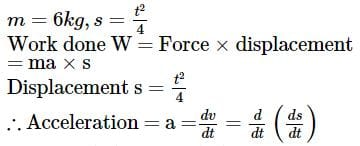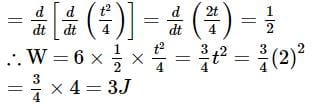BITSAT Physics Test - 3 - Question 38

The physical quantity which has the dimensional formula M1T⁻3 is

Detailed Solution for BITSAT Physics Test - 3 - Question 38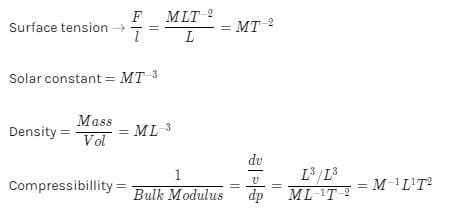BITSAT Physics Test - 3 - Question 39

A long spring when stretched by x cm has a potential energy V. On increasing the stretching to nx cm, the potential energy stored in the spring is

BITSAT Physics Test - 3 - Question 40

Two identical light waves, propagating in the same direction, have a phase difference δ . After they superpose the intensity of the resulting wave will be proportional to

## BITSAT Mock Tests Series & Past Year Papers

2 videos|15 docs|70 tests
 Use Code STAYHOME200 and get INR 200 additional OFF Use Coupon Code
Information about BITSAT Physics Test - 3 Page
In this test you can find the Exam questions for BITSAT Physics Test - 3 solved & explained in the simplest way possible. Besides giving Questions and answers for BITSAT Physics Test - 3, EduRev gives you an ample number of Online tests for practice

## BITSAT Mock Tests Series & Past Year Papers

2 videos|15 docs|70 tests